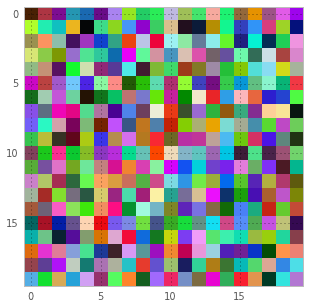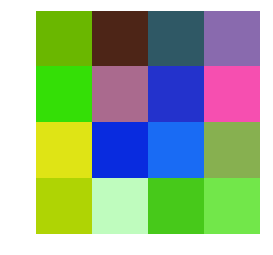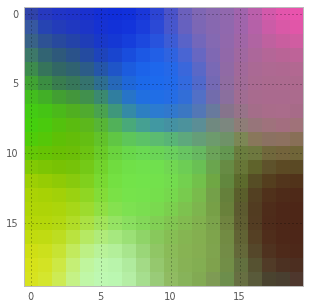# A Kohonen Map in Python (optimized by Numba)¶

Below is an implementation of a Kohonen map (also known as a self-organizing map).
Briefly, it's a technique for mapping a high-dimensional space onto a much lower one. You might say, "Well, principal components analysis can do that! Why do I need anything else?"
PCA does find a projection from a high-D space down to a simpler one, but it does so linearly. Meaning, it can only rotate, scale, add and subtract dimensions. Self-organizing maps are free to do much weirder transformations when compressiong dimensionality.

I needed a Kohonen map for my research, so I wrote this, and now I'm sharing it with you.

Enjoy!

• Alex Wiltschko
In :
import numpy as np
from numba.decorators import jit as jit
np.random.seed(1)


### Define helper functions¶

These guys below are the core of the Kohonen map algorithm. They've been numba-ized for speed.
What's interesting is that they're still relatively readable (quite readable for how fast they are).

In :
def scale(start, end, position_between_zero_and_one):
return double(start + position_between_zero_and_one * (end - start))

In :
nd0 = numba.double
nd1 = numba.double[:]
nd2 = numba.double[:,:]

@jit(arg_types=[ nd2, nd1, nd1 ])
def find_winner(som_weights, this_sample, abs_weight_distances):
winner_idx = 0
winner_distance = 1.0e100
num_nodes, num_dims = som_weights.shape

for i in range(num_nodes):
abs_weight_distances[i] = 0.0
for j in range(num_dims):
abs_weight_distances[i] += (((this_sample[j] - som_weights[i,j]) ** 2.0) ** 0.5)

In :
@jit(arg_types=[ nd1, nd2, nd0, nd2, nd0, nd0 ])
def update_weights(this_sample, som_weights, winner_idx, grid_indices, learning_rate, learning_spread):

winner_x = grid_indices[winner_idx,0]
winner_y = grid_indices[winner_idx,1]

num_nodes, num_dims = som_weights.shape
for i in range(num_nodes):
grid_distance = ((winner_x - grid_indices[i,0]) ** 2.0) + ((winner_y - grid_indices[i,1])**2.0)
dampening = e ** (-1.0 * grid_distance / ( 2.0 * learning_spread**2.0))
dampening *= learning_rate

for j in range(num_dims):
som_weights[i,j] += dampening * (this_sample[j] - som_weights[i,j])


### Setup parameters and allocate arrays¶

In :
# Generate some random colors for good times sake and what not have a good time yeah?
X = np.random.random((16,3))

# Some initial logistics
n_samples, n_dims = X.shape

# Construct the grid indices (2D for now)
# The grid indices are stored in a (numNodePoints x numDimensionsToGrid) array.
# It's easier to handle a list of the grid indices than it is to do anything otherwise.
grid_size = (20,20)
num_nodes = grid_size*grid_size
raw_grid = np.mgrid[0:grid_size, 0:grid_size]
grid_indices = np.zeros((num_nodes, len(grid_size)), dtype='d')
grid_indices[:,0] = raw_grid.ravel()
grid_indices[:,1] = raw_grid.ravel()

# Set parameters
num_iterations = 200
learning_rate_initial = 0.5
learning_rate_final = 0.1

# Allocate the weight distances
abs_weight_distances = np.zeros((num_nodes,), dtype='d')

# Initialize the som_weights
som_weights = np.random.random((num_nodes, n_dims))


### Show off the initialized map¶

In :
the_som = np.reshape(som_weights, (grid_size, grid_size, n_dims))
figure(figsize=(5,5))
imshow(the_som);

figure(figsize=(4,4))
axis('off')
numRows = 4
imshow(X[:,np.newaxis,:].reshape(numRows,X.shape/numRows,3))

Out:
<matplotlib.image.AxesImage at 0x11630db10>### Train the map¶

In :
import time
start = time.time()
for i in range(num_iterations):

# Pre-calculate the number of iterations (which will never be so impossibly large as to not store the indices)
idx = np.random.randint(0, n_samples, (num_iterations,))

# Pick a random vector
this_sample = X[idx[i],:]

# Figure out who's the closest weight vector (and calculate distances between weights and the sample)
find_winner(som_weights, this_sample, abs_weight_distances)
winner_idx = np.argmin(abs_weight_distances)

# Calculate the new learning rate and new learning spread
normalized_progress = float(i) / float(num_iterations)
learning_rate = scale(learning_rate_initial, learning_rate_final, normalized_progress)

# Update those weights
update_weights(this_sample, som_weights, winner_idx, grid_indices, learning_rate, learning_spread)

print "Training %d iterations on a %d-square grid took %f seconds" % (num_iterations, grid_size, time.time() - start)

Training 200 iterations on a 20-square grid took 0.033242 seconds


### Show off the finished Kohonen map¶

In :
the_som = np.reshape(som_weights, (grid_size, grid_size, n_dims))
figure(figsize=(5,5))
imshow(the_som);

figure(figsize=(4,4))
axis('off')
numRows = 4
imshow(X[:,np.newaxis,:].reshape(numRows,X.shape/numRows,3))

Out:
<matplotlib.image.AxesImage at 0x115ead390>